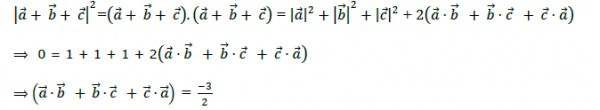# if a,b,c are three unit vectors such that a + b + c = 0 , then find the value of a.b + b.c + a.c ??

12 years ago

a+b+c=0,(a+b+c)^2=0,a2+b2+c2+2(a.b +b.c+a.c)=0,a2+b2+c2=3,therefore a.b+b.c+a.c= -(3/2)

12 years ago

why is a^2 + b^2 + c ^2 = 3??

7 years ago
Consider a,b,c as complex numbers which can be represented in the cartesian plane similar to vectors.
Let a=cosx + i sinx
b=cosy + i siny
c=cosz + i sinz
all the above complex numbers have modulus 1.
since a+b+c=0
hence sigma(cosx) + i sigma(sinx)=0
hence real and imaginary both parts are separately zero.
a^2+b^2+c^2=cos2x + cos2y + cos2z+ i(sin2x + sin2y + sin2z)=0
expand (a+b+c)^2=0 to get the result
6 years ago
a + b + c=0 Therefore ,(a+b+c)^2 =0Therefore (mod of a)^2 +(mod of b)^2 + (mod of c)^2 +2 (a.b + b.c + c.a) =0As a, b, c are unit vectors , square of their mod will be equal to oneHence 1 + 1+1 + 2 ( a.b+ b.c + c.a)=0 Therefore be equating, we get,a.b+ b.c + c.a = -3/2
5 years ago
a+b+C=0
(a+b+C)² =0
|a|² + |b|² + |c|² +2(a.b +b.c + c.a)=0
As it is given in the question that a, b, C are the unit vectors. Therefore, |a|=|b|=|c|=1.
So,
1+1+1+2(a.b + b.c + c.a)=0
2(a.b + b.c + c.a)= -3
a.b + b.c + c.a = -3/2Yash Chourasiya